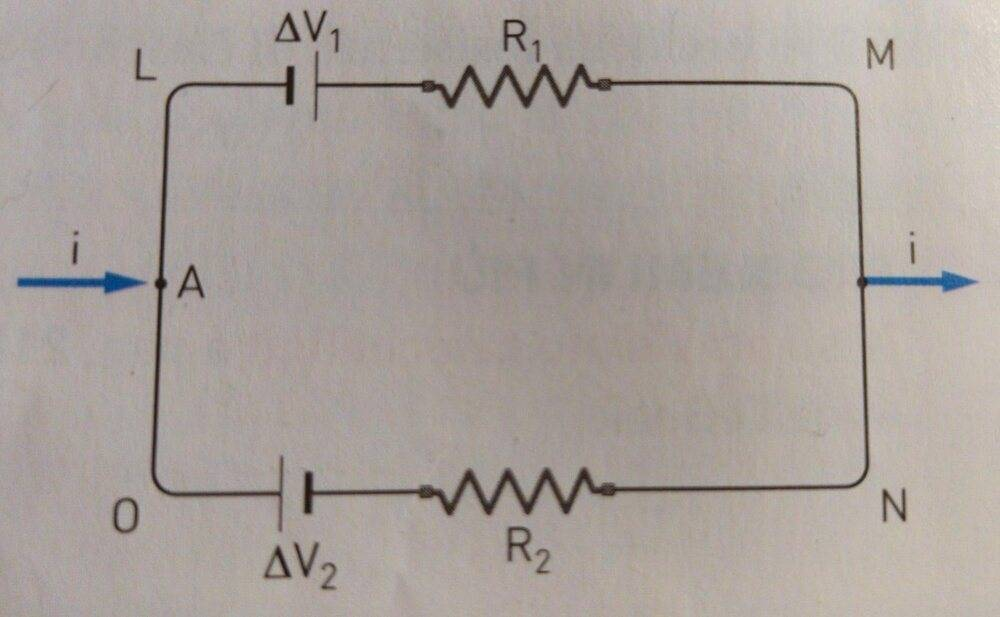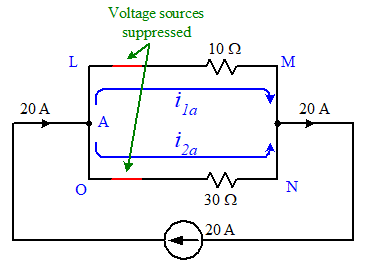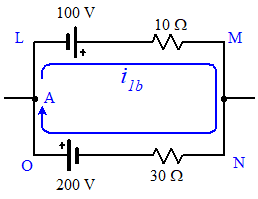# Using Kirchhoff's laws on this current division problem

• curiosissimo
curiosissimo
Homework Statement
We have a circuit (photo below) with 2 voltage generators and 2 resistors. In the node A the current intensity ##i=20A##. We know that ##ΔV1 = 100V##, ##ΔV2 = 200V##, ##R1 = 10Ω##, ##R2 = 30Ω##. We have to get the verse and the value of the currents ##i1## in the branch LM and ##i2## in the branch. ON. The exercise tells us to set the direction of travel clockwise.
Relevant Equations
Kirchhoff lawsThe main problem is the verse of the 2 currents ## i1 ## and ## i2 ## . I think they both go clockwise, so in the node A we should have ##i1+i2=20A##. Now let's apply the second law (going clockwise). So ##ΔV1-R1*i3 - R2*i4 + ΔV2=0##, where ##i3## and ##i4## are the currents which pass through ##R1## and ##R2##. But why aren't ##i3## and ##i4## equal to ##20A##? According to the solution, ##i1## is positive, while ##i2## is negative. So is ##i2## going counterclockwise? It doesn't make sense, it can't.

•Delta2

Homework Helper
Gold Member
You are already given that I1 and I2 are the currents through branches LM and ON. Why define I3 and I4 additionally?

You are correct that I1+I2=20, but only if you denote the directions of I1 and I2 correctly. For this equation to be correct:
In what direction does I1 flow in LM? In what direction does I2 flow in ON?
What will be the KVL equation if you trace the loop clockwise (A-L-M-N-O-A)?

•curiosissimo
curiosissimo
You are already given that I1 and I2 are the currents through branches LM and ON. Why define I3 and I4 additionally?

You are correct that I1+I2=20, but only if you denote the directions of I1 and I2 correctly. For this equation to be correct:
In what direction does I1 flow in LM? In what direction does I2 flow in ON?
What will be the KVL equation if you trace the loop clockwise (A-L-M-N-O-A)?

I1 flows clockwise as well as I2. In fact I2 can't go counterclockwise from O to N, because after O we have V2 and at first there is the positive pole. But I2 follows the negative one.

curiosissimo
I get the solution if the verse of I2 is counterclockwise, but why? It should go clockwise following the negative pole.

Homework Helper
Gold Member
In fact I2 can't go counterclockwise from O to N, because after O we have V2 and at first there is the positive pole. But I2 follows the negative one.
You cannot judge that without knowing the exact circuit.
You can assume that I2 flows from N to O (clockwise), but then I1+I2=0 does not hold at A.

You should be consistent with signs and polarities in KCL and KVL equations.
If your assumed current direction/voltage polarity is wrong, you get a negative answer (assuming you treat reference direction/polarity positive), which is still correct.

•curiosissimo
curiosissimo
You cannot judge that without knowing the exact circuit.
You can assume that I2 flows from N to O (clockwise), but then I1+I2=0 does not hold at A.

You should be consistent with signs and polarities in KCL and KVL equations.
If your assumed current direction/voltage polarity is wrong, you get a negative answer (assuming you treat reference direction/polarity positive), which is still correct.
Why I1+I2 = 0 if I2 is clockwise? I miss this point

curiosissimo
I mean, the generators are oriented in the same way, so I1 and I2 go in the sale direction

Mentor
I mean, the generators are oriented in the same way, so I1 and I2 go in the sale direction
Only if you consider only the current due to those two voltage sources alone. The current entering at ##A## will divide through the circuit and either add to or subtract from those currents depending upon their relative directions.

If you've been introduced to the superposition theorem you might be able to get a better feel for why the net current in each branch can be flowing from left to right in the circuit.

If you suppress the two voltage sources leaving only the current flowing into ##A## and out the other side of the circuit, you have something like this:You should be able to calculate both ##i_{1a}## and ##i_{2a}## readily enough using basic current divider properties.

Next, suppress the current source and leave the voltage sources and use KVL to find the clockwise loop current:If ##i_{2a}## is greater than ##i_{1b}## then it (##i_{1b}##) will be "overwhelmed" in terms of net current flow direction through the lower branch.

Last edited:
•cnh1995 and curiosissimo
curiosissimo
Only if you consider only the current due to those two voltage sources alone. The current entering at ##A## will divide through the circuit and either add to or subtract from those currents depending upon their relative directions.

If you've been introduced to the superposition theorem you might be able to get a better feel for why the net current in each branch can be flowing from left to right in the circuit.

If you suppress the two voltage sources leaving only the current flowing into ##A## and out the other side of the circuit, you have something like this:

View attachment 269937

You should be able to calculate both ##i_{1a}## and ##i_{2a}## readily enough using basic current divider properties.

Next, suppress the current source and leave the voltage sources and use KVL to find the clockwise loop current:

View attachment 269938

If ##i_{2a}## is greater than ##i_{1b}## then it will be "overwhelmed" in terms of net current flow direction through that branch.
Ah I got it. I wasn't considering that the current was entering the circuit "outiside".
What about there's no current entering from outside? I mean, what if I have to find the total current in the circuit with the same problem data (except for i=20)?

Mentor
Ah I got it. I wasn't considering that the current was entering the circuit "outiside".
What about there's no current entering from outside? I mean, what if I have to find the total current in the circuit with the same problem data (except for i=20)?
Then you do only "step two" in the superposition approach above; only the voltage sources are present and you have a single closed loop. You'll find the single loop current and compare its direction to the given current directions that you're trying to find (say from L to M and from O to N).

•curiosissimo
curiosissimo
Thank you all

Homework Helper
Gold Member
Why I1+I2 = 0 if I2 is clockwise? I miss this point
At node A, I2 and 20A will be "entering" and I1 will be "leaving".
So, if you apply KCL at node A, the equation will be I1-I2=20.
Just didn't want to leave any question unanswered from my side.Perhaps you have understood this now after gneill's explanation.

•curiosissimo
curiosissimo
At node A, I2 and 20A will be "entering" and I1 will be "leaving".
So, if you apply KCL at node A, the equation will be I1-I2=20.
Just didn't want to leave any question unanswered from my side.Perhaps you have understood this now after gneill's explanation.
Ok I got it, thanks :)